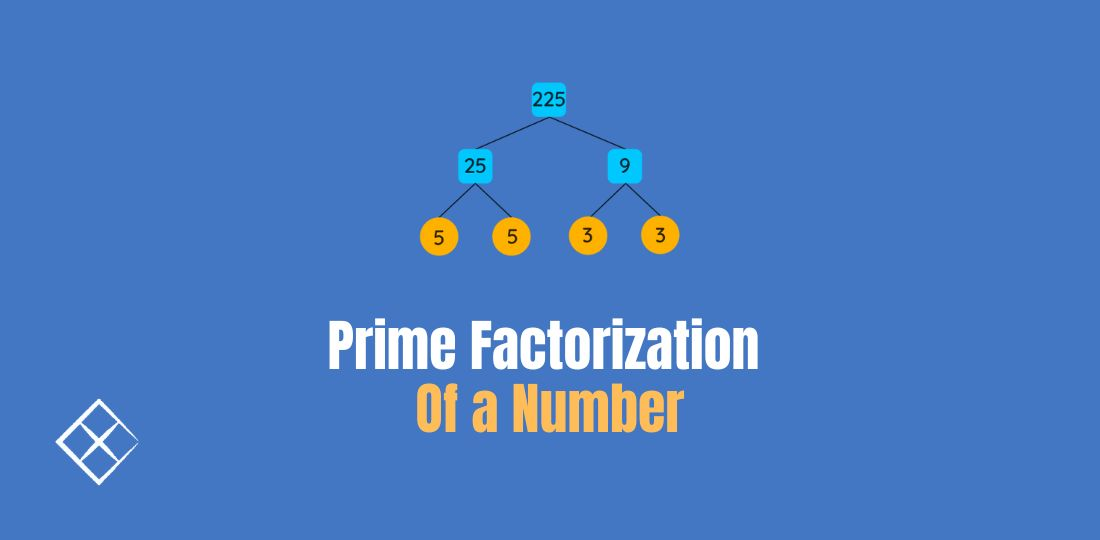# Prime Factorization of a Number# Problem Statement

How do we find all the prime factors of a given number?

For example, when `n = 12`, the output should be `[2, 3]`. Notice that `1, 4, 6, 12` are also factors of 12 but they aren’t prime and therefore should be excluded from the output.

## Solution

The following solution is straightforward:

``````def get_prime_factors(n: int) -> List[int]:
prime_factors = []
prime = 2
while n > 1:
while n % prime == 0:
if not prime_factors or prime != prime_factors[-1]:  # deduplicate
prime_factors.append(prime)
n //= prime
prime += 1
return prime_factors
``````

## 2 Keys Keyboard

This problem is an application of the prime factorization algorithm:

``````def minSteps(n: int) -> int:
prime = 2
steps = 0
while n > 1:
while n % prime == 0:
steps += prime
n //= prime
prime += 1
return steps
``````

## Count Ways to Make Array With Product

This problem is a more involved application of the prime factorization algorithm. To understand the below algorithm, please review https://en.wikipedia.org/wiki/Stars_and_bars_(combinatorics)#Theorem_two_2.

``````from math import comb

class Solution:
def waysToFillArray(self, queries: List[List[int]]) -> List[int]:
res = []
for n, k in queries:
powers = self.total_power(k)
ans = 1
for p in powers:
ans *= self.count_sum_up(n, p)
res.append(ans)
return res

def total_power(self, n: int) -> int:
res = []
prime = 2
while n > 1:
power = 0
while n % prime == 0:
power += 1
n //= prime
if power > 0:
res.append(power)
prime += 1
return res

def count_sum_up(self, n: int, total: int) -> int:
return comb(n + total - 1, n - 1)
``````

Note that the above code will not be accepted by leetcode. I’ve omitted two details: 1) the answers should modulo `10^9 + 7` and 2) we need to memoize methods `total_power` and `count_sum_up`. The omissions are intentional so the attention can be focused on the main logic.

One of the constraints of the problem states that each `k` does not exceed `10^4`. We can further optimize by precomputing all primes less than or equal to `10^4`:

``````class Solution:
def waysToFillArray(self, queries: List[List[int]]) -> List[int]:
self.primes = self.get_primes_at_most(10000)
res = []
for n, k in queries:
powers = self.total_power(k)
ans = 1
for p in powers:
ans *= self.count_sum_up(n, p)
res.append(ans)
return res

def total_power(self, n: int) -> int:
res = []
prime = 2
for prime in self.primes:
if prime > n:
break
power = 0
while n % prime == 0:
power += 1
n //= prime
if power > 0:
res.append(power)
return res

def count_sum_up(self, n: int, total: int) -> int:
return comb(n + total - 1, n - 1) % (pow(10, 9) + 7)

def get_primes_at_most(self, n: int) -> List[int]:
primes = []
nums = [True] * (n + 1)
for i in range(2, n + 1):
if nums[i]:
primes.append(i)
nums[i] = False
for j in range(i * i, n, i):
nums[j] = False
return primes
``````

Method `get_primes_at_most` is an implementation of Sieve of Eratosthenes.